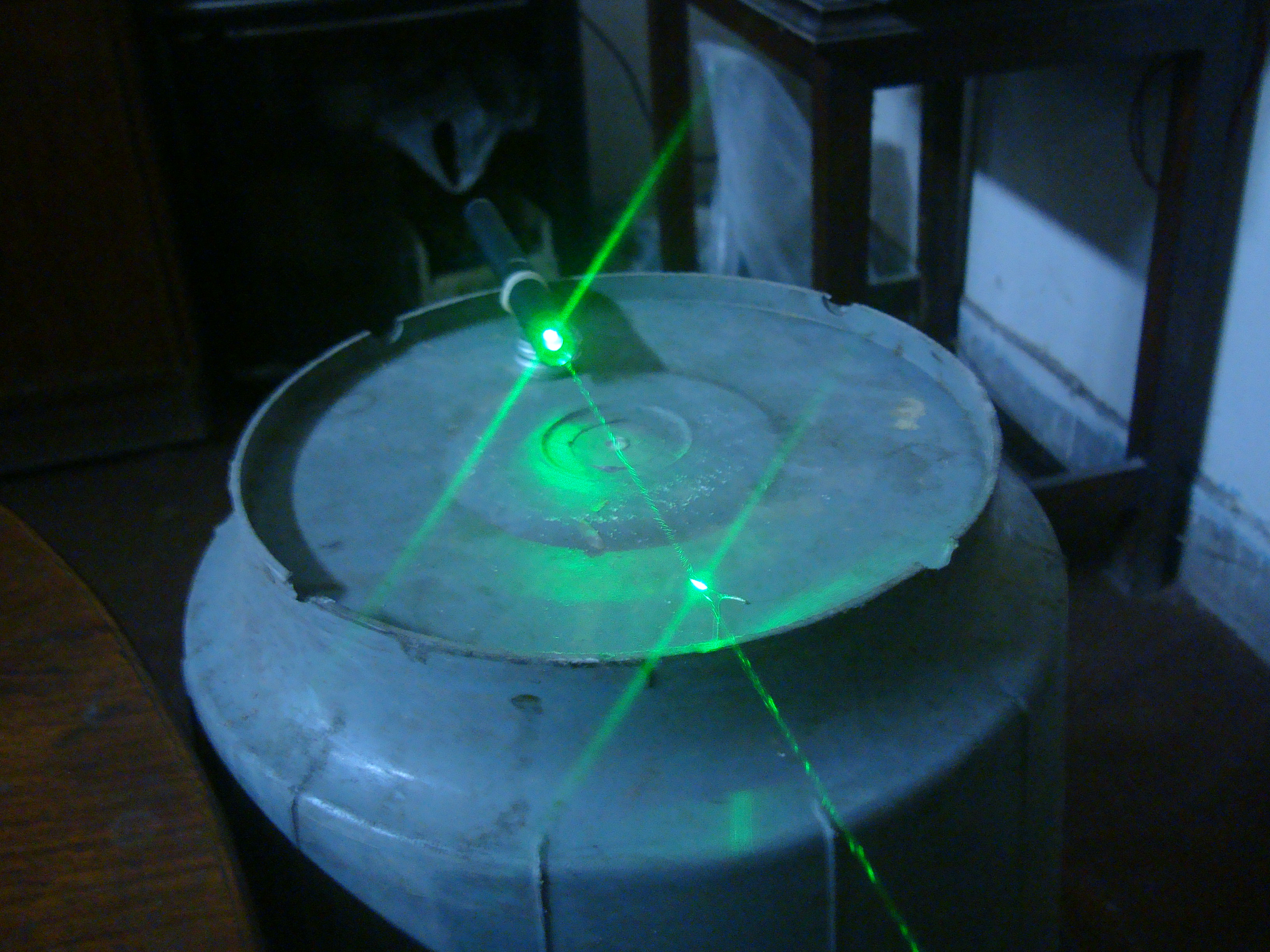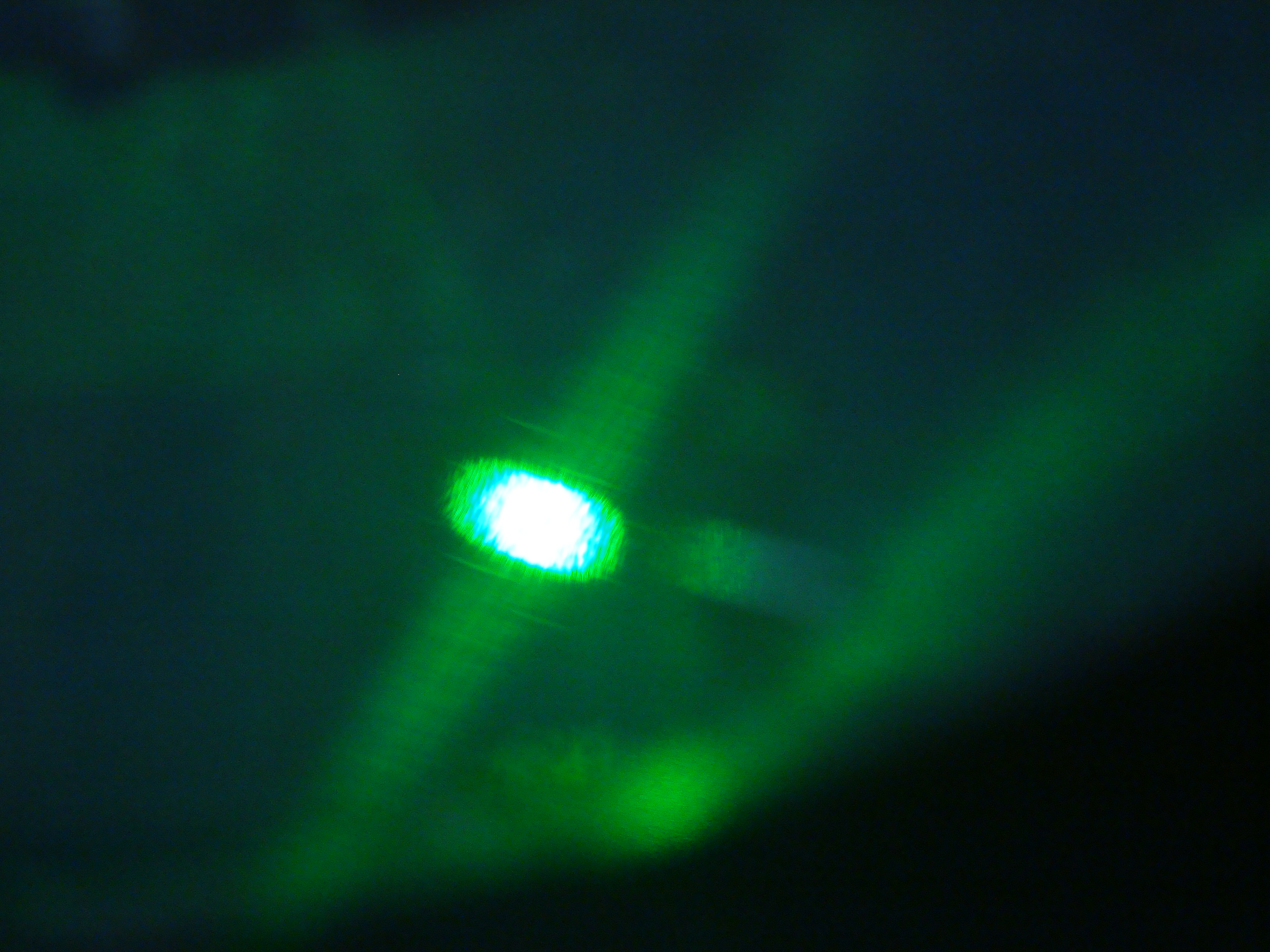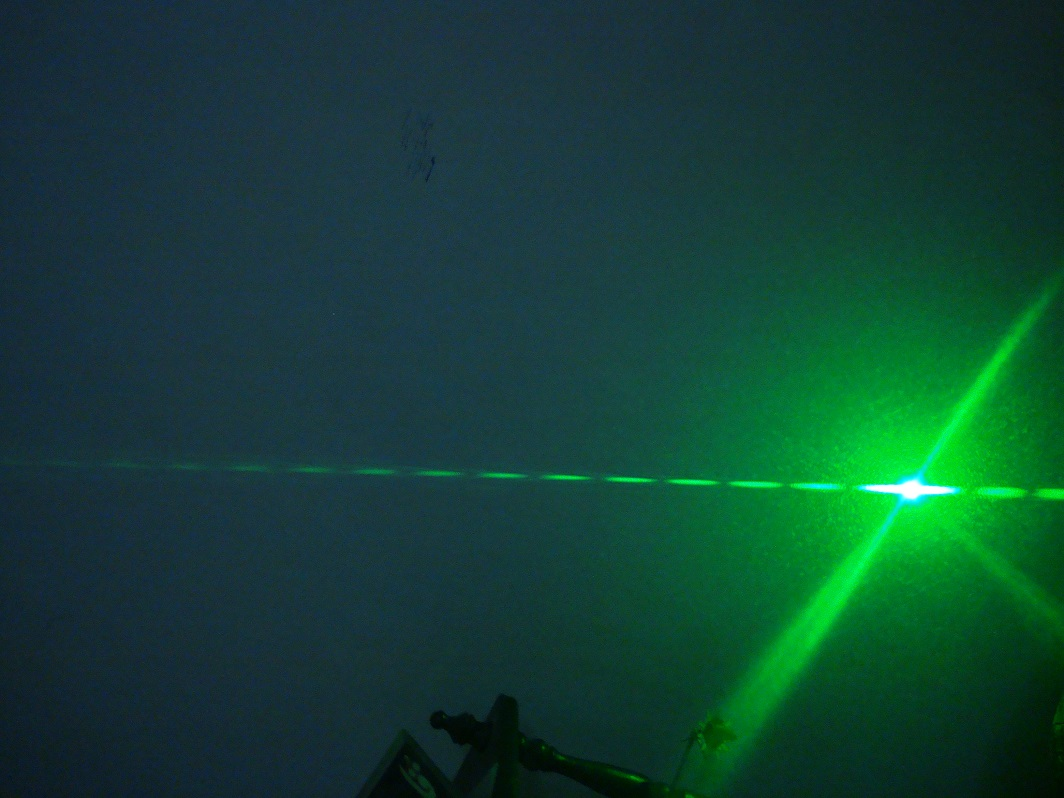## Wednesday, 25 June 2014

### deBroglie Relations and the scale of Quantum Effects, light waves as particles (b)

ii. a microwave operates at roughly $2.5 GHz$ at a max power
of $7.5 *10^9 erg/s$
How many photons per second can it emit? What about a
low-power laser ($10^4 erg/s$ at $532nm$)?

Ans:
1.First for the microwave,
The energy of one such microwave photon is $E = h v$
where the frequency v is $2.5*10^9 Hz$
so:
$E = 6.63*10^{-27}*2.5*10^9$

$E = 1.657*10^{-17}erg$

This is the energy, we have the power in erg/s which is $7.5*10^9erg/s$, to find the number of photons emitted we need to divide the power by the energy:

$\text{number of photons }=\frac{P}{E}$

$\text{number of photons }=\frac{7.5*10^9}{1.657*10^{-17}}$

$\text{number of photons }= 4.5265*10^{26}$

2.Now for the Laser,
Since now except the frequency, we have the wavelength we can substitute the formula of frequency in our energy formula:
$E=h*(\frac{c}{\lambda})$
where the frequency v is $2.5*10^9 Hz$
so:
$E = 6.63*10^{-27}*2.5*10^9$

$E = 1.657*10^{-17}erg$

$E=6.63*10^{-27}*(\frac{299,792,458}{532*\frac{1}{1*10^{9}}})$

$E=3.73*10^{-12}erg$

Now divide it with power:

$\frac{1*10^4}{3.73*10^{-12}} = 2.7*10^{15}photons$

### Quantum micrometer experiment, how to find the width of your hair

HI! Yesterday I did an experiment to find the width of my hair using my green laser. The method is to hit the laser beam on the hair, the light passing above the hair will be like going through a slit, and the light passing below the hair will be like going though a slit. So in other words it is a type of double slit experiment. By using the effect of wave-particle duality of a photon, and the pattern of interference, we can calculate the distance between the two slits, or in other words the width of my hair.This is the setup of my so called lousy apparatus :P. I have supported the laser on the bucket using a stack of 4 coins, to adjust the beam to hit the hair. the hair is supported by a wire, which I have bended here and there for support. The wall where the interference pattern forms is 6.72meters away from the hair.This was the bucket with the laser and hair on it. the light flare ahead is where the beam hits the hair.A close up of the hair and the beam hitting it.So this was the interference pattern produced 6.72 meters away from my hair. The number of these fringes is 18, you can only see 15 in this pic, there are three more to the right. you have to count till where you cant even see anything. The length from the center fringe to the 18th fringe is 0.87meters, note all these values down.

To do this calculation there are two formulas which I had.

they are formulas to find the distance between the two slits, which will be the width of my hair.

the formula is $d = \frac{n l \lambda}{x}$
and
$d=\frac{n*\lambda*\sqrt{x^2+l^2}}{x}$ which is the same as the upper formula, but it uses the distance from the hair to the outer edge of the interference pattern. Im going to use both to find more accurate results.

in these formulas:
$\lambda$ = wavelength of laser in meters.= $532*10^{-9}m$
$n$ = the number of fringes. = $18$
$l$ = distance from hair to wall = $6.72m$
$x$ = distance from center of pattern to farthest countable fringe = $0.87m$

plugging these in the first formula we get:

$d = \frac{n l \lambda}{x}$
$d = \frac{18*6.72*532*10^{-9}}{0.87}$
$d = 0.000074m$

so using this fromula I get 74microns.

Now plugging in in the second formula we get:

$d = \frac{n*\lambda*\sqrt{x^2+l^2}}{x}$
$d = \frac{18*532*10^{-9}*\sqrt{(0.87)^2+(6.72)^2}}{0.87}$
$d = 0.0000746$

so using this formula I get 74.6microns.

Now I will take the average of these values I get 74.3 microns!!

For more accuracy, the lasers intensity should be high, distance between the hair and wall should be long, and the wavelength of the laser should be low.

violet lasers are best!

## Monday, 23 June 2014

### deBroglie Relations and the Scale of Quantum Eﬀects, Light waves as particles (a)

(a) Light Waves as Particles:
The Photoelectric eﬀect suggests that light of frequency ν can be regarded as
consisting of photons of energy $E=h v$, where $h = 6.63*10^{-27}erg*s$

i. green light has a wavelength in the range of 532nm. What are the
energy and frequency of a photon of green light?

Ans:
First to find the frequency we can use the equation: $c=v \lambda$
putting in the values:
$299,792,458 = v*532$

$v = \frac{299,792,458}{532*\frac{1}{1*10^9}}$ mid calculation unit conversion from nm to m

$v = 5.45077*10^{14}Hz$

so the frequency is $545.077 THz$

Now we can use the above equation of $E=h v$ to find the energy of the photon
so:
$E = 6.63*10^{-27}*545.077*10^{14}$

$E = 3.614*10^{-10} erg$

AKA $36.14\text{ nano ergons}$